# Texas Go Math Grade 5 Lesson 15.4 Answer Key Analyze Bar Graphs

Refer to our Texas Go Math Grade 5 Answer Key Pdf to score good marks in the exams. Test yourself by practicing the problems from Texas Go Math Grade 5 Lesson 15.4 Answer Key Analyze Bar Graphs.

## Texas Go Math Grade 5 Lesson 15.4 Answer Key Analyze Bar Graphs

Unlock the Problem

A bar graph is useful for comparing and analyzing data.

Sometimes you can make a prediction based on data. A prediction is a reasonable guess about what may happen, and may end up being true or false.

Example 1.
Mrs. Marzipan sold pretzels in a snack bar in a city park.
She recorded the number of pretzels sold each day for 5 days.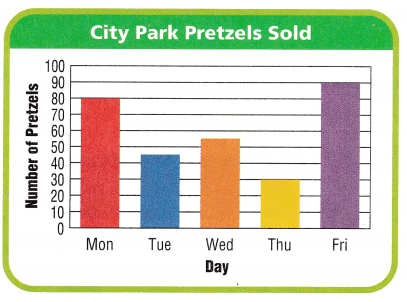• For how many days did Mrs. Marzipan record the number of pretzels she sold?

• What is being compared in this bar graph?

A. Draw a conclusion.

On which day was the greatest number of pretzels sold?
Conclusion: The greatest number of pretzels was
sold on ____

B. Make a prediction.
On which day next week will
Mrs. Marzipan sell the most pretzels?
Prediction: Next week, Mrs. Marzipan will
sell the most pretzels on ___

Example 2
Solve a multi-step problem.

Students earn $3 for every magazine subscription they sell. How much more money does Aaron earn than Carla? Step 1 Use the graph to find the number of subscriptions Aaron and Carla each sell. Aaron: ___ subscriptions Carla: ____ subscriptions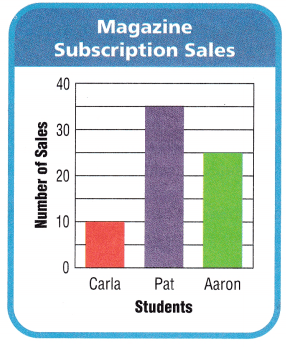Step 2 Multiply to find the amount of money they each earn. Aaron: ___ ×$3 = $____ Carla: ___ ×$3 = $____ Step 3:$___ – $___ =$____
So, Aaron earns $___ more selling magazine subscriptions than Carla. Share and Show Use the bar graph for 1-2. Lesson 15.4 Bar Graph Go Math Answer Key Grade 5 Question 1. How many more clamshells than scallop shells does Barb have?number of clamshells: ____ number of scallop shells: ____ ___ – ___ = ___ ___ clamshells Answer: Question 2. What is the total number of seashells in Barb’s collection? Answer: Math Talk Mathematical Processes Describe how the bars on the graph would look if Barb had 2 times as many conch shells as clamshells. The marbles in a bag are red, blue, yellow, and green. Albert draws 20 marbles from the bag and records the results In a bar graph. Use the bar graph for 3-4. Question 3. Connect When you combine the number of two colors of marbles that Albert draws, it is equal to the total number of another color of marbles he draws. Write an equation using color words to represent this relationship.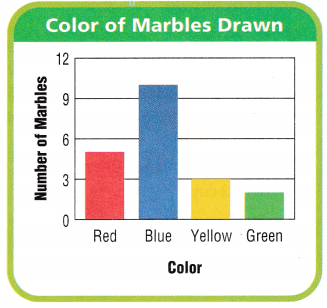Answer: Question 4. What color in the bag most likely outnumbers any other color in the bag? Justify your answer. Answer: Use the graph for 5-6. Question 5. Multi-Step An article in the school newspaper states that Hector received more than half the votes in the election. Is this statement correct? Explain.Answer: Go Math 5th Grade Analyzing Bar Graphs Lesson 15.4 Question 6. H.O.T. Analyze Twenty students who did not vote in the election. If these students had voted for candidates other than Hector, how might the results of the election be different? Give three different possibilities.Answer: Daily Assessment Task Use the bar graph for 7-9. Fill in the bubble completely to show your answer. Question 7. The teachers at Marty’s school are in a pasta-cooking contest. The bar graph shows the amounts of sauce they use in their recipes. What is the difference between the greatest and least amounts of sauce? (A) 7 cups (B) 2 cups (C) 1 cup (D) 6 cupsAnswer: Question 8. Analyze Who could fill more than one 1-quart container with their sauce? Hint: 1 quart = 4 cups (A) Steve (B) Earl (C) Ana (D) Jaime Answer: Question 9. How much sauce do all the teachers use in their recipes? (A) 5 cups (B) 7 cups (C) 15 cups (D) 14 cups Answer: Texas Test Prep 5th Grade Math Analyze Bar Graph Lesson 15.4 Question 10. The bar graph shows the number of animals on a farm. The animals eat hay every day. Each horse eats 15 pounds; each cow eats 30 pounds; and each pig eats 1 pound. How much hay do all the farm animals eat in 1 day?(A) 46 pounds (B) 636 pounds (C) 591 pounds (D) 33 pounds Answer: ### Texas Go Math Grade 5 Lesson 15.4 Homework and Practice Answer Key A football team uses a bar graph to record and compare the scores for the first four games. Use the bar graph for 1-3. Question 1. How many more points did the team score in game 4 than in game 2? ______ Answer:Question 2. How many points did the team score in the first three games? ____ Answer: Question 3. What is the total number of points the team scored? ____ Answer: Problem Solving Students participate in a park clean-up event on Saturday. The principal records the attendance in a bar graph. Use the graph for 4-5. Question 4. Tyler says the fifth graders have more than twice as many participants as the second graders. Is Tyler correct? Explain. Answer: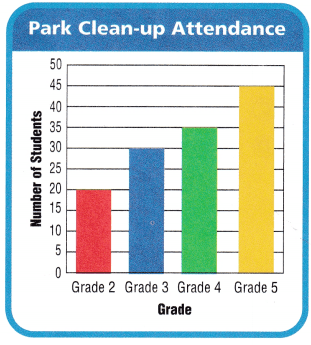Go Math 5th Grade Answer Key Graph and Analyze Relationships Question 5. ‘What if 27 more third graders arrive after lunch to help with the clean up? What is the difference between the number of students in the grade with the greatest attendance and the number of students in the grade with the least attendance? Explain. Answer: Texas Test Prep Fill in the bubble completely to show your answer. Question 6. The bar graph shows the number of hours each person worked at the movie theater on Friday. Al earns$8 an hour, Bob earns $10 an hour, and Elena earns$9.50 an hour. How much will the theater owner pay all three workers on Friday?(A) $158 (B)$168
(C) $171 (D)$181

A kennel cares for dogs while their owners are on vacation. The graph shows the amount of food each dog receives each day based on his weight and activity level. Use the graph for 7-9.

1 pint = 2 cups
1 quart = 2 pints
1 gallon = 4 quarts

Question 7.
Which dog is fed more than 1 quart but less than $$\frac{1}{2}$$ gallon of food each day?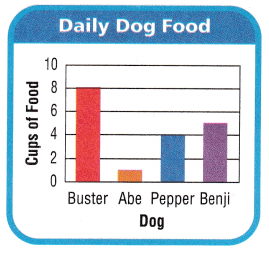(A) Buster
(B) Abe
(C) Pepper
(D) Benji

Question 8.
Multi-Step How much food is Pepper fed in one week?
(A) 7 pints
(B) 7 quarts
(C) 25 cups
(D) 7 gallons